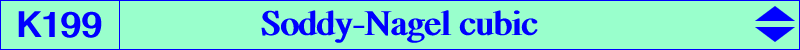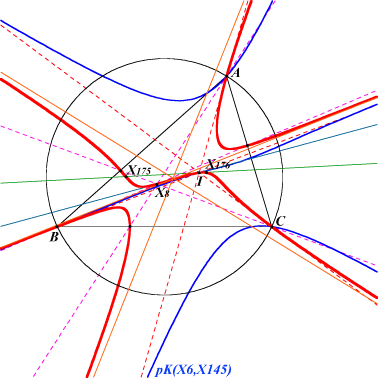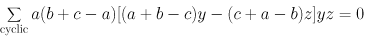X(1), X(8), X(40), X(175), X(176), X(188), X(280), X(483) CPCC or H-cevian points, see Table 11The Soddy-Nagel cubic K199 is pK(X9, X8). The pivot is the Nagel point X(8) and it passes through the Soddy centers X(175), X(176). See also K032 and K200. Its asymptotes are parallel to those of K1086 = pK(X6, X145). It meets the circumcircle at the same points as pK(X6, X3869). Its isogonal transform is K632 = pK(X604, X1) and its isotomic transform is pK(X85, X75). More generally, any pK with pivot X(8) and pole on the line through X(2), X(7), X(9), ... contains the Soddy centers. *** The A-vertex osculating circle is centered at {0,-b,c}. As such, the centers of the three vertex osculating circles lie on the antiorthic axis. (Peter Moses, 2019-11-23)